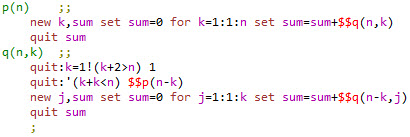Recursion

Once upon a time I audited a computer science course CS-101.  One day the professor wrote on the blackboard, "Recursion: See Recursion."  I thought he had made up the joke. However, later, I found that many people had thought up this clever definition, or variants of it.  Wikipedia attributes the earliest version to the book Software Tools, by Kernighan and Plauger (1976).

The professor's example was the factorial function. For non-negative integers N, if N = 0 the factorial is 1, if N > 0, the factorial is N times Factorial(N-1).

For laboratory work in that course, we used the PL/C language (based on IBM's PL/1).  At the time of this course, around the same time as Kernighan and Plauger's book, I had not yet heard of databases, or of object oriented programming--or fractals.  In short, I had no idea of the power and reach of recursion.  Nevertheless, the idea had an immediate fascination.

When the time came to select a term project (counting extra points for students who were taking the course for credit), I submitted a problem that would exercise resursive programming.

The problem was to count ties in a made-up variant of the game of pool.  In mathematics, this is known as a partition problem.  However, I did not know anything about partitions at the time.My step-son Greg had a toy pool table similar to the one pictured. The balls were about the size of marbles and the sticks were proportionately reduced in size. We did not know the rules of pool, so invented them. Two players took turns, each attempting to sink a ball by hitting it with the white ball. At the end we added the numbers on the balls that each player had sunk. Occasionally this scoring method resulted in a 60-60 tie, an outcome which immediately suggested the puzzle, “In how many different ways can the game be tied?” In other words, how many distinct subsets of the integers 1, 2, 3, …, 15 (where each number can be used only once in each subset) yield a sum of 60.

I do not intend to work out the ties-at-pool problem here—it was presented in print, a long time ago.,, Nevertheless, to convey the idea, consider that the highest numbered pool ball is 15. Four times 15 is 60 (a tie score), but there is only one ‘15’ ball, as there is one of each number 1, 2, …, 15. Thus, if aiming to make a tie score we use the four highest numbered balls, 15 + 14 + 13 + 12, there will be a deficit of 6. In other words 15 + 14 + 13 + 12 + 6 yields a tie score.

Clearly the four highest numbered balls plus any combination of low numbered balls that sum to 6 also make a tie. Thus, 6, 5 + 1, 4 + 2, and 3 + 2 + 1 all work. However, 4 + 1 + 1 does not work, because there is only one ‘1’ ball. Partitions that satisfy the pool puzzle are qualified in the way that each number contributing to the sum can be used only once. Unrestricted partitions do not have this qualification. There are 11 unrestricted partitions of the number 6.

6    5    4    4    3    3    3    2    2    2    1

1    2    1    3    2    1    2    2    1    1

1         1    1    2    1    1    1

1         1    1    1

1    1

1

To more fully appreciate the recursive nature of parititons, a good exercise would be to work out on paper the number of partitions of 7 or another small number. Although I failed to solve the ties-at-pool problem while auditing Computer Science 101, I did solve it when learning MUMPS a few years later.

The recursive (or should I say “truly twisted”) MUMPS function to compute the number of unrestricted partitions p(N) has its tail in its mouth in more than one way. By that I mean that the function p(N) calls q(N,K), which in turn calls both p and q.When commands are spelled out, MUMPS code reads like pseudo-code.  Thus it is probably clear how function p works. p(N) simply sums the numbers of contributing partitions (subsets) whose maximum element is K, for each K. Deciphering function q is a little trickier. This function encodes the process one would carry out to enumerate partitions using pencil and paper, substituting recursive calls at exactly those points where the process repeats.

To conclude, while this minimalistic example does not begin to convey the true power and scope of recursion, as applied in computer programming or computer science, the example might serve to illustrate how recursion in a sense amplifies the power of computational steps, in order to achieve a result that is not as easily computed in other ways.

 I have this book, thanks to my friend Bain H.  The colophon says, "This book was set in Times Roman and Helvetica Regular by the authors, using a Graphic Systems phototypesetter driven by a PDP-11/45 running under the Unix operating system."  Ah!, the good old days.
 I never got the problem to work in PL/C.  The cycle time between submitting a batch of cards and recieving back the error listing was too long.  I had a day job at the time!
Wondrous Numbers and Other Diversions, Creative Computing, volume 2, Number 4, April 1985, pages 99-101.

MUMPS Recursion Using the NEW command, MUG Quarterly, volume 15, Number 2, Summer 1985, pages 65-67.

The number of ties in a game played with 15 balls is 722.  Note that for any outcome of the game, tie or not, exchanging the identity of players yields a complementary outcome. Thus, counting complementary outcomes as distinct, the number of ties is always even.
 The number 7 has 15 unresetricted partitions.